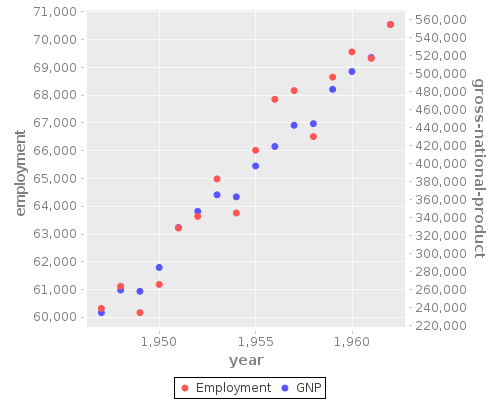### Plot Multiple Y Axis with Incanter & Clojure

#### July 2, 2018    ClojureIncanterData ScienceMisc

Incanter doesn’t have a built in way to add an additional Y-axis.

``````(defn merge-y-axis
"Merge the Y axis of two line / time series charts. The former chart will have
the left Y axis, and the latter will have the right."
[^JFreeChart chart ^JFreeChart chart-to-merge]
(let [^XYPlot plot (.getPlot chart-to-merge)]
(doto ^XYPlot (.getPlot chart)
(.setRangeAxis 1 (.getRangeAxis plot))
(.setDataset 1 (.getDataset plot))
(.mapDatasetToRangeAxis 1 1)
(.setRenderer 1 (.getRenderer plot)))
(-> (.getPlot chart)
(.getLegendItems)
chart))
``````

Then you can

``````~/\$ lein repl
user=> (use '(incanter core datasets charts))
user=>
;; Get built in employment dataset
(let [[employment _ gross-national-product _ _ _ year] (to-vect (trans (to-matrix data)))]
;; Plot year / employment on the first axis
(doto (scatter-plot year employment :series-label "Employment" :legend true)
;; Merge in another chart that plots year / gross national product
(merge-y-axis (scatter-plot year gross-national-product :series-label "GNP" :legend true)
(view))))
``````

and you’ll get